# Knowledge Base

## Solution 34713: Finding the Reduced Row Echelon Form of a Matrix on the TI-84 Plus C Silver Edition Graphing Calculator.

### How do I find the reduced row echelon form of a matrix on a TI-84 Plus C Silver Edition?

To find the reduced row echelon form of a matrix, input the matrix, exit the matrix editor, and then paste the rref() function on the home screen with the name of the matrix. The example below will demonstrate this procedure.

For Example: Find the rref() of matrix A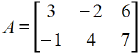To enter the matrix:

1) Press [2nd] then [MATRIX]
2) Scroll to Edit, and press [ENTER] on the very first option. This will enable you to edit matrix A.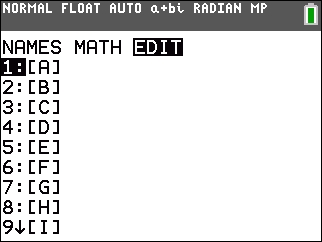3) Input the dimensions of the matrix by pressing , [ENTER], , and then [ENTER].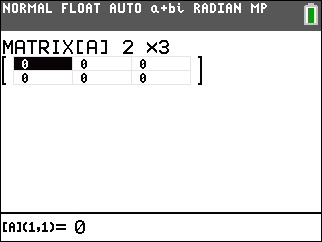4) Input the matrix entries, pressing enter after each entry.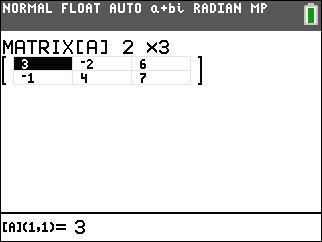5) Exit the matrix editor by selecting [2nd] >> [QUIT].

To find the reduced row echelon form using the rref( function:

6) Press [2nd] then [MATRIX].
7) Scroll to "MATH" by pressing the right arrow key one time.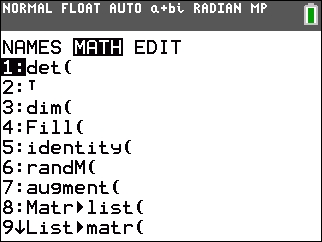8) Scroll down until you see "rref(", which is the function for reduced row echelon form. Press [ENTER] on that option, which then pastes the function onto your home screen.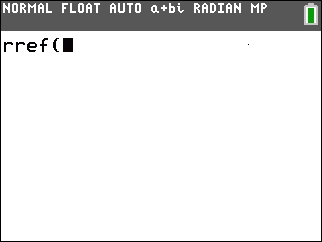9) Press [2nd] then [MATRIX]  [ENTER], which pastes Matrix A into the rref command. Lastly, close the parenthesis by pressing [)], press [ENTER], and you'll have your answer.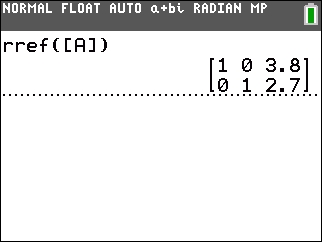Please see the TI-84 Plus C Silver Edition guidebook for additional information.